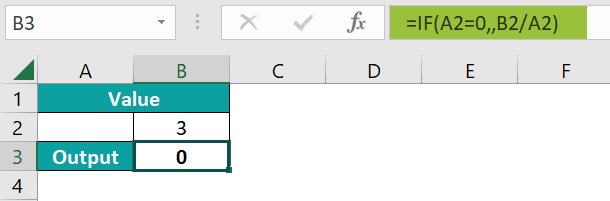# #DIV/0! Excel ErrorArticle byPriyanka Bera## What Is A #DIV/0! Error In Excel?

The Division Error or the #DIV/0! Error in Excel is the error that occurs when we divide any cell value or cell reference by zero or a blank cell, or if any cell reference of a formula contains the #DIV/0 error.

For example, we will apply the #DIV/0! Error to calculate the error values as the output.

Select cell A2, enter the formula =10/0, and press “Enter”.

The result is returned as ‘#DIV/0!’ error’, as shown above.

###### Key Takeaways
• The #DIV/0! error is caused by dividing by zero but can also be generated due to other formulas referencing cells that display the #DIV/0! error.
• It is useful because it tells that something is missing or unexpected in the data.
• It also appears when data is entered but is not yet complete or any blank cell because it is not yet available.
• If a blank cell is left in the data and division calculation is to be performed, Excel considers the blank cell value as 0, and the evaluated result will generate #DIV/0! Error.

### Scenarios Of Getting “#DIV/0!” In Excel

During Excel calculations, we get “#DIV/0!” in the following scenarios,

• Dividing by Zero.
• Summing Cells.
• AVERAGE Function.

#### #1 – Dividing by Zero

We will perform calculations and generate the #DIV/0! Excel Error for the Dividing by Zero scenario.

In the table, the data is,

• Column A contains the Values.
• Column B contains the Output.

The procedure to get the #DIV/0! Error is as follows:

Select cell B2, enter the formula =A2/A3, and press the “Enter” key.

The result is a “#DIV/0!” error, as shown above.

#### #2 – Summing Cells

We will perform calculations and generate #DIV/0! Excel Error for the Summing Cells scenario.

In the table, the data is,

• Column A contains the Values.
• Column B contains the Output.

The steps to get the #DIV/0! Error are as follows:

• Step 1: Select cell B2, and enter the formula =SUM(A2:A7), to calculate the sum of the values.
• Step 2: Press the “Enter” key. The result is a “#DIV/0!” error, as shown below.

#### #3 – AVERAGE Function

We will perform calculations and generate #DIV/0! Excel Error for the AVERAGE excel Function scenario.

In the table, the data is,

• Column A contains the Values.
• Column B contains the Output.

The steps to get the #DIV/0! Error are as follows:

• Step 1: Select cell B2, and enter the formula =AVERAGE(A2:A7), to calculate the average of the values.
• Step 2: Press the “Enter” key. The result is a “#DIV/0!” error, as shown below.

### How To Fix “#DIV/0!” Error In Excel?

We can fix the “#DIV/0!” error in Excel using the IFERROR(), ISERROR(), and IF() functions.

#### #1 – Remove #DIV/0! Error Using IFERROR

We will use the IFERROR function in Excel to remove the #DIV/0! error.

In the table, the data is,

• Column A contains the Values.
• Column B contains the Output.

The steps to remove the #DIV/0! Error using the IFERROR() are as follows:

• Step 1: Select cell B2, enter the formula =SUM(A2:A3)/0, press the “Enter” key, and drag the formula from cell B2 to B3 using the fill handle. The results are “#DIV/0!” error, as shown below.
• Step 2: Let us fix the result in cell B3, to understand the difference.

Select cell B3, enter the formula =IFERROR(SUM(A2:A3)/0,“Sum is Divided by 0”), and press the “Enter” key. The result is the “Sum is Divided by 0”, as shown below.

#### #2 – Remove #DIV/0! Error Using IF

We will use the IF function in Excel to remove the #DIV/0! error.

In the table, the data is,

• Column A contains the Values.
• Column B contains the Output.

The steps to remove the #DIV/0! Error using the IF() are as follows:

• Step 1: Select cell D2, enter the formula =C2=B2/C2, and press the “Enter” key. We will get an error pop-up, click “OK”. The result is a “#DIV/0!” error, as shown below.
• Step 2: Let us fix the result in cell D2, to understand the difference.

Select cell D2, enter the formula =IF(C2=0,0,B2/C2), press the “Enter” key, and drag the formula from cell D2 to D3 using the fill handle. The results are “0”, and “0.8”, as shown below.

#### #3 – Remove #DIV/0! Error Using ISERROR

We will use the ISERROR function in Excel to remove the #DIV/0! error.

In the table, the data is,

• Column A contains the Values.
• Column B contains the Output.

The steps to remove the #DIV/0! Error using the ISERROR() are as follows:

• Step 1: Select cell B2, enter the formula =A2/A3, and press the “Enter” key. The result is a “#DIV/0!” error, as shown below.
• Step 2: Next, select cell B3, enter the formula =ISERROR(B2), and press the “Enter” key. The result is “TRUE”, as shown below.

### Find All Cells With #DIV/0! Excel Error

The steps to Find All Cells With #DIV/0! Excel Errors are as follows:

1. We must first open the file where we want to remove the #DIV/0! Errors. Then, press the “CTRL+F” keys, i.e., the shortcut keys to open the “Find and Replace” dialog box in Excel.
1. Click on the “Options” button, which shows additional options.
1. In the ‘Find what:’ box, type #DIV/0!
1. From the ‘Within’ drop-down with the Sheet or Workbook options, select the “Sheet” option.
1. From the ‘Look in’ drop-down, select the ‘Values’ option.
1. Click on “Find All”, which will find all the cells that have the #DIV/0! error, as shown below.

### Important Things To Note

• We get a #DIV/0! error in the AVERAGE function when the dataset does not have any numerical value.
• The #DIV/0! error occurs in the SUM excel function when the selected cell range has one or more of the cell values as the #DIV/0! value in it.
• We can avoid getting a #DIV/0! Error by making sure that a division calculation doesn’t have the divisor as 0 or the excel cell reference for the divisor is not a blank cell or 0.

1. DIV/0! Error and Blank cells?

Let us consider an example where we get a #DIV/0! Error due to blank cells.

We will first divide the values, and generate the #DIV/0! Excel Error when the reference cell is passed is blank.

In the table, the data is,
• Column A & B contains the Values.
• Row 3 contains the Output.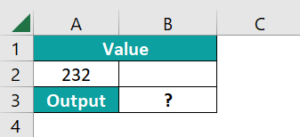The procedure to get the #DIV/0! Error with a blank cell is,

Select cell B3, enter the formula =A2/B2, and press the “Enter” key.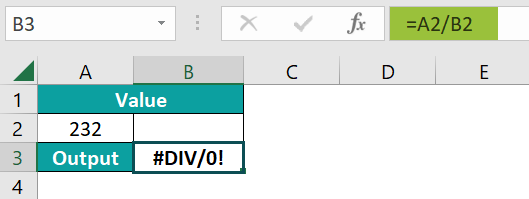The result is a “#DIV/0!” error, as shown above.

2. What does the #DIV/0! in Excel mean?

The #DIV/0! in Excel means that there is a division error in the formula or the cell reference, such as a number divided by zero, or the dataset has a blank cell, or the result of the formula is zero, which is the number getting divided.

3. How to show a Zero instead of #DIV/0! Error?

We can display a Zero instead of #DIV/0! Error as follows:

First, create a data table, as shown below.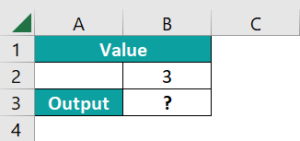Next, choose cell B3 → select the “Formulas” tab → go to the “Function Library” group → click the “Insert Function” option on the far left, as shown below.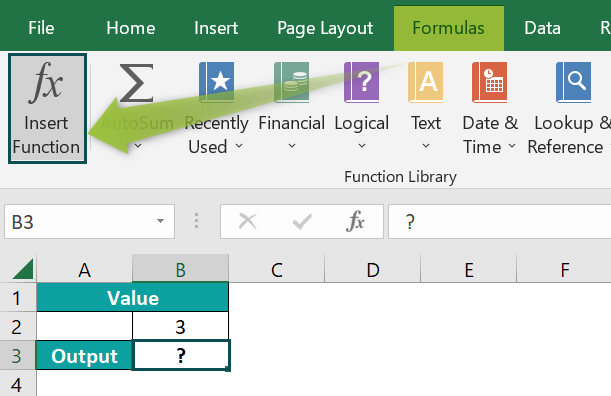The “Insert Function” window appears. In the “select a category:” drop-down, select the “Logical” option, as shown below.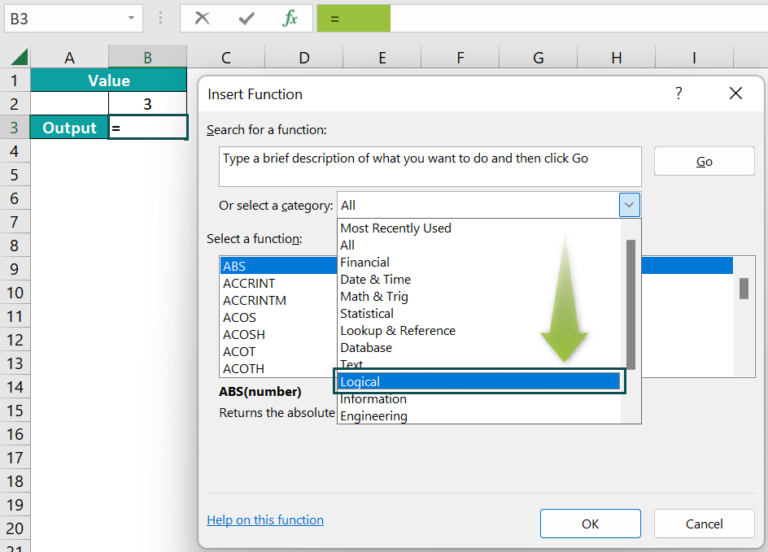Now, the options change in the “Select a function:” group, then select the IF function, and close “OK”, as shown below.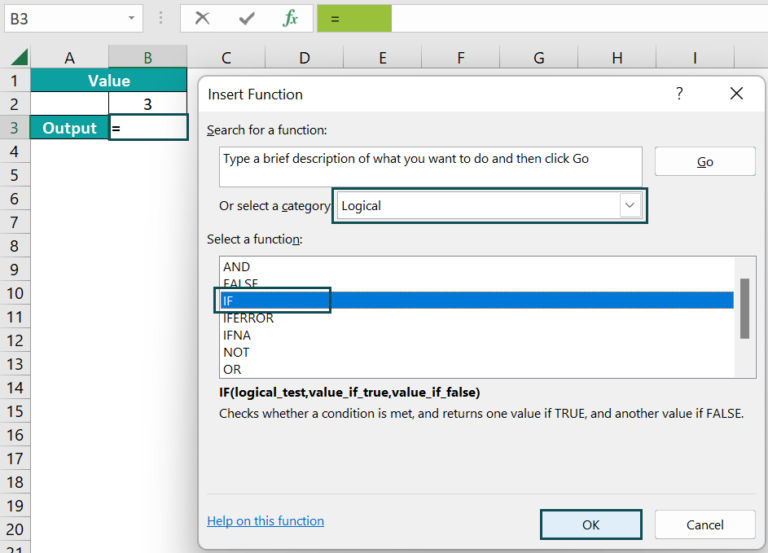Once we click “OK” in the “Insert Function” window, the Function Arguments window appears.

Enter in the Logical_test field as A2=0, the Value_if_true field blank, the Value_if_false field as B2/A2, and click “OK”, as shown below.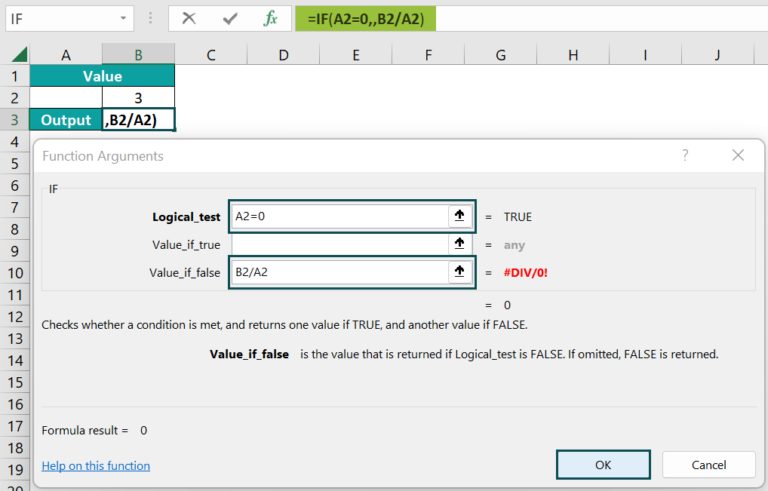So the complete formula will be =IF(A2=0,,B2/A2). We will get the result ‘0’, as shown below.